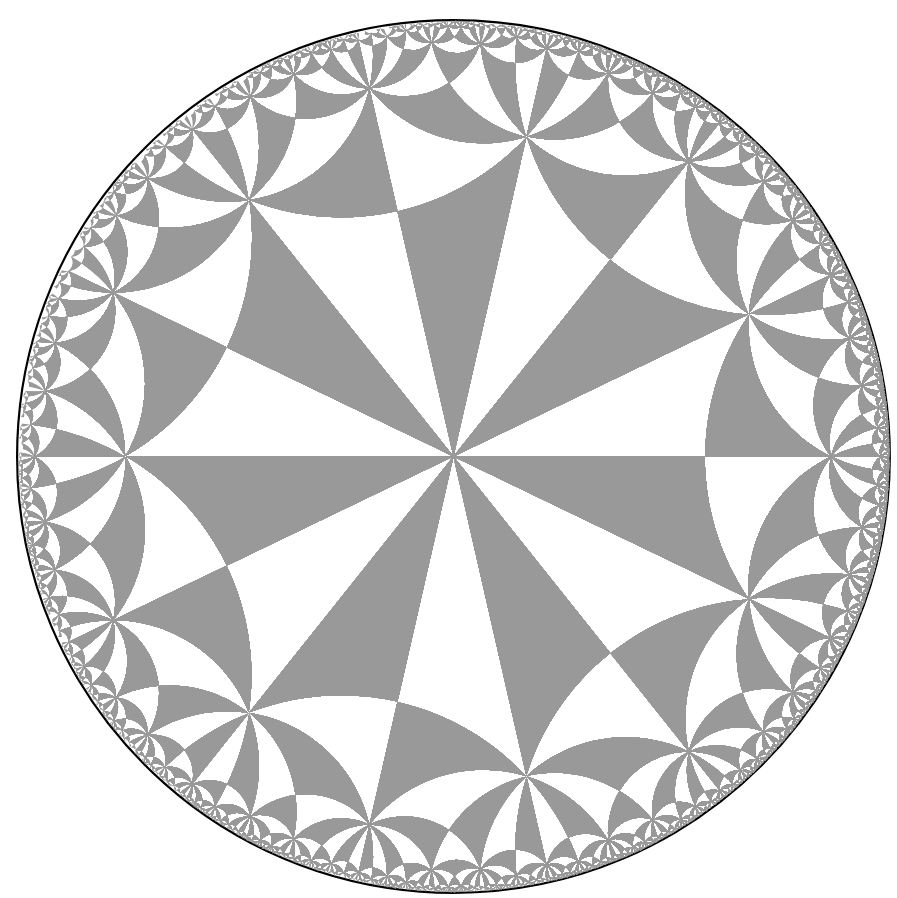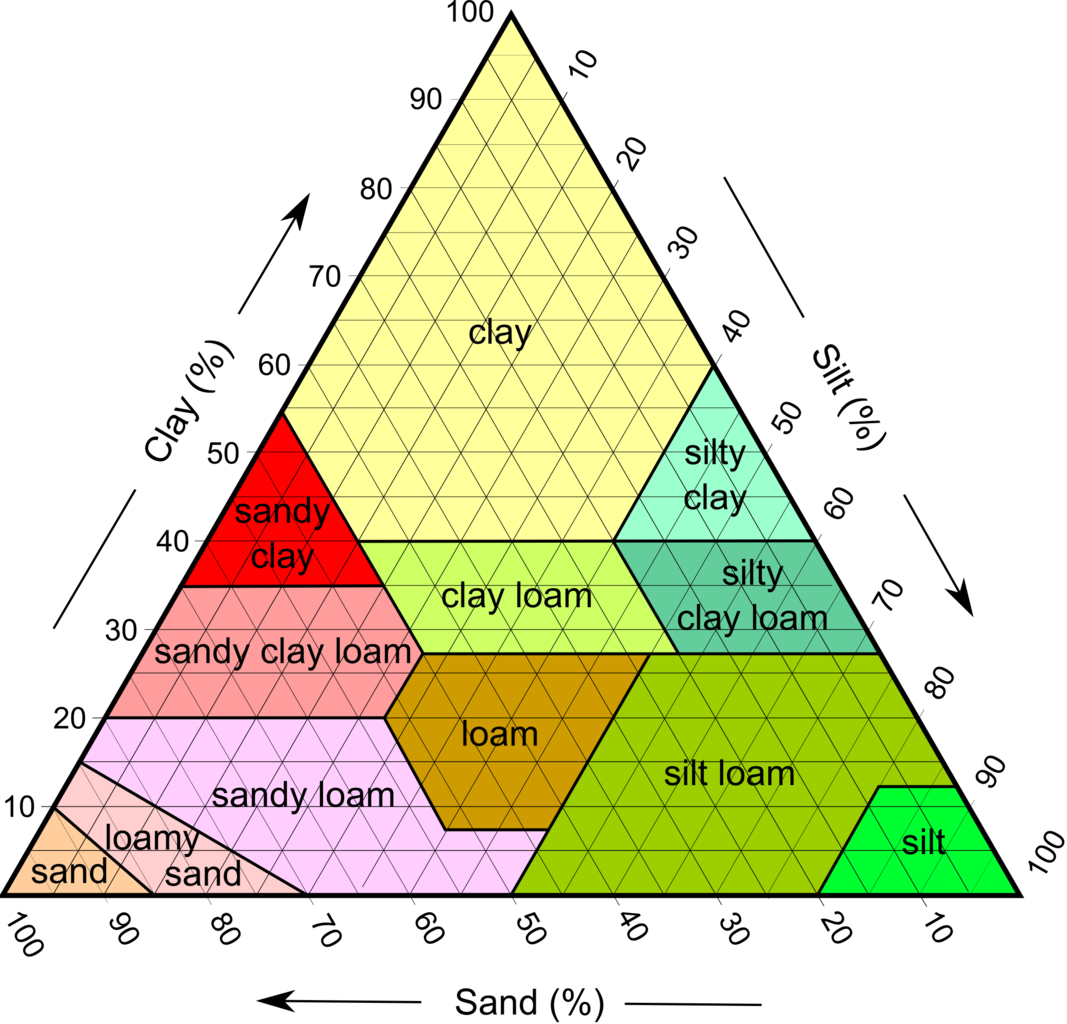# More morsels

1. Vehicular verbs. We say we're on a bus, a train, a plane, a boat, but in a car, rocket, submarine. What's the rule?

I think the implicit rule is that if you must walk to reach your seat, you're on the vehicle. If the seat is imminently "right there", then you're in the vehicle. This nicely distinguishes the passenger jet from the slim fighter jet, generalizes to new vehicles, and suggests that you might invent a mixed-seat vehicle with a combination of both types of seating.

2. RPG damage. In a certain game, your weapon destroys each object $$i$$ in a certain characteristic number of hits $$k_i$$. Each time you upgrade your weapon, it decreases the number of required hits by one. Can you account for this observed behavior by supposing each object has a certain fixed number of hit points $$C_i$$ and the weapon with $$n$$ upgrades deals a certain number of damage $$\delta_n$$ each hit?

Yes: these constraints describe a system of linear inequalities: $\delta_j (k_i - j - 1) < C_i \leq \delta_j(k_i - j)\qquad i=1,\ldots,m; j=0,\ldots,n$

These imply that for any $$a$$ and $$b$$ in the range, $\delta_a (k_i - a - 1) < \delta_b(k_i - b)\qquad i=1,\ldots,m.$ When there are no upgrades $$(n=0)$$ or one upgrade $$(n=1)$$ possible, the system is always solvable.

When there are two upgrades $$(n=2)$$, there's an interesting limit to the allowed range of $$k_i$$ values: $$k_{max} < 3(k_{min}-1)$$. And additional upgrades further tighten this constraint.

3. An empty regular expression. You should be able to program a regular expression for any regular language. What about the empty language—can you make a regular expression that doesn't match any string at all?

Here's one way: /\$^/. Isn't that cute?

4. Crossing in pairs There's a puzzle where four musicians are trying to cross a bridge in the dark using only one flashlight. Based on the instruments they carry, each person can cross in, respectively, 1min, 2min, 5min, and 10min. Up to two people can cross the bridge at the same time, going the slower person's speed; one of the bridge crossers must always carry the flashlight. Find a solution that brings everyone across the bridge in under 18min.

It's interesting to see how the optimal strategy changes depending on the musicians' transit times: for example, the quickest strategy is different when the numbers are [1, 2, 5,10] versus [1, 4,5, 10]. You can prove that if the numbers, in increasing order, are [A,B,C,D], the quickest strategy is determined by how A+C compares to 2B.

You can do an algebraic treatment for n musicians, finding regions of n-dimensional space where the optimal strategy changes. http://logical.ai/puzzle.pile/crossings/

And in fact, you can prove that you can always get the optimal result by greedily sending the two slowest people across, either using a "quick escort" (1,k),(1),(1,k-1),(1) or a "one-two ratchet" (1,2),(2),(k,k-1),(1)—whichever is faster. Here, 1,2,..,n order the people from fastest to slowest, and (A,B) denotes a trip where A and B travel together.

5. Drawing like Escher. Escher's mesmerizing tesselation drawings are hyperbolic tesselations. They're made out of repeating congruent triangles that live on a curved rather than flat surface, so when the design is projected onto flat paper, they appear to be different sizes and made out of curved lines.Surprisingly, you can build these symmetric designs by starting with a single triangle and generating new triangles using mirror reflections (to capture symmetry). The triangles are all constructed in 3D space, then the resulting diagram is projected onto a 2D paper.

• You determine the design by specifying two parameters: $$p$$, the number of triangles surrounding the origin, and $$q$$, the number of triangles meeting at each vertex. To fit on a hyperbolic curved surface, the parameters must satisfy the equation $$1/p + 1/q < 1/2$$. (If instead they're equal to 1/2, the pattern fits on a flat plane. If they're less than 1/2, it fits on a sphere.)
• The Weierstrass space consists of all points (u,v,w) on the 3D hyperboloid surface $$u^2 + v^2 - w^2 = 1$$.
• The primal triangle (as I call it) is the fundamental repeating unit of your design. In Weierstrass space, its vertices are at:

• [0,0,1]
• $$\langle 1, \frac{\sin{(2\pi/p)}}{1+\cos{(2\pi/p)}}, \frac{\sinh{(2b)}}{-1 + \cosh{(2b)}}$$
• $$\langle -1 + \cosh{(2b)}, 0, \sinh{(2b)}\rangle$$

where $$\cosh{(b)}= \cos(\pi/q) / sin(\pi/p)$$ and so consequently $$\cosh{(2b)} = 2\cosh^2(b) - 1$$ and $$\sinh{(2b)} = \sqrt{\cosh^2{2b}-1}$$.

• To make new triangles from our primal triangle, we will reflect it (in 3D space) over one of its three sides. The vertices of the resulting new triangle will still satisfy the Weierstrass space equation.

The three reflections are:

• Reflect over the perpendicular bisector: $$\begin{bmatrix}1 & &\\&-1&\\ &&1\end{bmatrix}$$
• Reflect over the other leg: $$\begin{bmatrix}-\cosh{2b} & &\sinh{2b}\\&1&\\-\sinh{2b} &&\cosh{2b}\end{bmatrix}$$
• Reflect over the hypotenuse: $$\begin{bmatrix}\cos{(4\pi/p)}&\sin{(4\pi/p)}&\\\sin{(4\pi/p)}&-\cos{(4\pi/p)}&\\&&1\end{bmatrix}$$
• Successively applying these three reflections will generate the entire tesselation. Of course, the full figure requires an infinite number of reflections—but paper has finite resolution, so you can stop after some finite number.
• Once you've collected all of the triangles, you can project them onto 2D space for drawing using the "Poincare transform" $$\langle u,v,w\rangle \mapsto \frac{1}{1+w}\langle u,v\rangle$$.

Note that straight lines in Weierstrass space become circular arcs in 2D space.

• Python code: http://logical.ai/worklife/2021%20week%2040%20oct/minimal_tessellate.py
6. Triangle plots. A soil texture diagram shows how soil is named based on how much silt, sand, and clay it contains:Note that every point within the triangle corresponds to a specific amount of silt, sand, and clay. The three variables have been compressed neatly into two dimensions using the constraint that the three percentages must all add up to 100%. Note how any two percentages uniquely determine a point within the triangle.

The general idea is what I call a triangle plot. When you have three variables $$A,B,C$$ that must add up to 100%, you can arrange them in a triangle plot as follows.

To read a point $$\langle x, y\rangle$$ off the 2D plot, you can use: \begin{align*}A(x,y) &= \frac{2}{\sqrt{3}}y \\ B(x,y) &= x - \frac{1}{\sqrt{3}}y\\ C(x,y) &= -x -\frac{1}{\sqrt{3}} y + 1\end{align*}

To write a point $$\langle a, b, c\rangle$$ onto the triangle, you can use any number of redundant equations:

\begin{align*} x=b-\frac{2}{3}a &\qquad y=\frac{2}{\sqrt{3}}a\\ x=\frac{3}{4}b+\frac{\sqrt{3}}{4}c&\qquad y=\frac{\sqrt{3}}{4}b - \frac{3}{4}c\\ x=2a+\sqrt{3}c &\qquad y=2a/\sqrt{3}\\ \end{align*}

And the plot occupies an equilateral triangle between $$\langle 0,0\rangle$$, $$\langle 1,0\rangle$$, and $$\langle 1/2, \sqrt{3}/2\rangle$$.

Of course you can make triangle plots when $$a,b,c$$ are not percentages but instead add up to some other fixed value; just multiply $$A,B,C$$ and divide $$a,b,c$$ by that value.

7. Dice polynomials. Any collection of polyhedral dice (as found in tabletop rpgs) can be represented as a one-variable polynomial, where the exponent denotes the type (number of sides) of each die, and the coefficient denotes how many dice have that type. For example, if you have three six-sided dice and one twenty-sided die, you'd represent it as the polynomial $$1d^{20} + 3d^6$$.

Note that by using d for the indeterminate, the usual dice notation (e.g. d20+3d6) emerges (!).

You can compute important properties of a dice roll by evaluating the polynomial. Specifically, note that numerical functions f(n) can be added together, multiplied by constants, and exponentiated (so f3(x) = f(f(f(x))), and f0(x) = x). Define three functions F(n)=1+n, G(n)=1, and H(n)=½+n.

Then for any dice polynomial P(d):

• The highest achievable value is given by P(F)(0)
• The lowest achievable value is P(G)(0),
• The expected value is P(H)(1/2).

And, for what it's worth, the variance is P(q)(-1)/12, where $$q(x) = (1+x) + 2 \sqrt{1+x}$$.

Date: 2022/Jan/6

Created: 2022-08-03 Wed 21:42

Validate